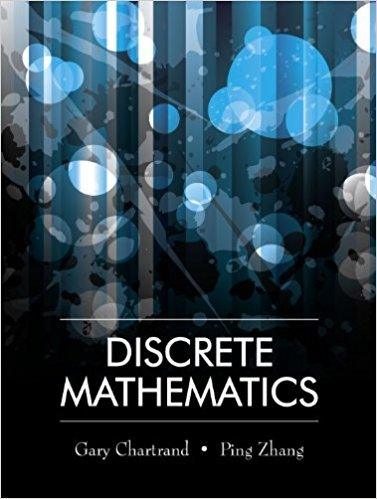×
×

# For a positive integer n, a total of 19n students have volunteered to serve on aISBN: 9781577667308 385

## Solution for problem 13 Chapter 8.5

Discrete Mathematics | 1st Edition

• Textbook Solutions
• 2901 Step-by-step solutions solved by professors and subject experts
• Get 24/7 help from StudySoup virtual teaching assistantsDiscrete Mathematics | 1st Edition

4 5 1 387 Reviews
25
3
Problem 13

For a positive integer n, a total of 19n students have volunteered to serve on a committee. Of these, 3n are freshmen, 6n are sophomores and 10n are juniors. A total of 9n students are wanted for the committee, namely 2n freshmen, 3n sophomores and 4n juniors. How many committees are possible? Express your answer in terms of factorials.

Step-by-Step Solution:
Step 1 of 3

L8 - 7 Recall One-Sided Limits Theorem. lim f(x)= L if and only if x→c  −2 x ≤−...

Step 2 of 3

Step 3 of 3

##### ISBN: 9781577667308

The full step-by-step solution to problem: 13 from chapter: 8.5 was answered by , our top Math solution expert on 03/13/18, 07:11PM. This textbook survival guide was created for the textbook: Discrete Mathematics, edition: 1. The answer to “For a positive integer n, a total of 19n students have volunteered to serve on a committee. Of these, 3n are freshmen, 6n are sophomores and 10n are juniors. A total of 9n students are wanted for the committee, namely 2n freshmen, 3n sophomores and 4n juniors. How many committees are possible? Express your answer in terms of factorials.” is broken down into a number of easy to follow steps, and 59 words. Since the solution to 13 from 8.5 chapter was answered, more than 209 students have viewed the full step-by-step answer. This full solution covers the following key subjects: . This expansive textbook survival guide covers 79 chapters, and 1714 solutions. Discrete Mathematics was written by and is associated to the ISBN: 9781577667308.

Unlock Textbook Solution